# Two pumps together

The first pump will fill the tank itself in 3 hours and the second one in 6 hours. How many hours will the tank be full if both pumps are worked at the same time?

t =  2 h

### Step-by-step explanation: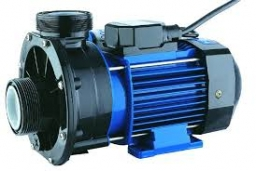Did you find an error or inaccuracy? Feel free to write us. Thank you!Tips to related online calculators
Looking for calculator of harmonic mean?
Looking for a statistical calculator?
Need help to calculate sum, simplify or multiply fractions? Try our fraction calculator.
Do you want to convert time units like minutes to seconds?

## Related math problems and questions:

• Two pumps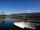The first pump fills the tank in 24 hours second pump in another 40 hours. For how long will it take to fill the tank if it operates both pumps at the same time?
• Two pumpsPump A takes twice as long to fill a tank as B. Together they take 2 hours to fill the tank. How long does each one take to fill the tank by itself?
• Three pumpsWe are filling the pool. The first pump would be filled in 12 hours, the second pump in 15 hours. If all three pumps were running at the same time, it would fill the pool for 4 hours. How long would the pool fill only with the third pump?
• PumpingThe tank is filled with a larger pump in 12 hours and with scond pump in 15 hours. For how long does the tank fill when both pumps are switched on at the same time?
• Three pumps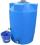The water tank is emptied with the first pump in 6 hours, with the second pump in 2 hours. In order to be emptied in 1 hour, all three pumps had to be started at once. How long will take tank be emptied by only the third pump?
• Three pumps together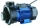One pump fills the tank in 1.5 hours, the second in 2 hours, and the third in 3 hours 20 minutes. How many minutes will the tank fill with three pumps if they work simultaneously?
• Five pumps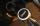Three same pumps fill the tank with 50400 liters of diesel in 7 hours. How many liters of diesel will it take in 4 hours if we add two more of the same pumps and pump them the same way? How much more (or less) will they get if we add 2 of the same pumps a
• Tank 9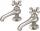The tank with volume V is filled with one pump for three hours and by the second pump for 5 hours. When both pumps run simultaneously, calculate: a) how much of the total volume of the tank is filled in one hour b) for how long is the tank full
• Pumps A and BPump A fill the tank for 12 minutes, pump B for 24 minutes. How long will take to fill the tank if he is only three minutes works A and then both pumps A and B?
• Pumps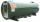6 pump fills the tank for 3 and a half days. How long will fill the tank 7 equally powerful pumps?
• Pumps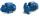Four identical pumps fill the tank in 40 hours. How many pumps would we have to use if we wanted to save 8 hours?
• 5 pumps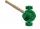Five pump to pump 3 hours 1600 hl of water. How long pumped same amount of water 4 pumps?
• Inlet minus outlet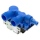Water flows into the tank through two pipes and flows out of the third pipe spontaneously. Pipe A itself would fill the tank in 3 hours, pipe B itself would fill in 4 hours, and pipe C would flow out in 12 hours. How long will the tank be full if all thre
• Tanker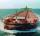An oil tanker can be emptied by the first pump in 4.9 hours. The second pump can empty a tanker in 8 hours. If the first pump started at 4:00 and the second 1.4 hour later, at what time will the tanker be empty?
• Pumps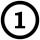First pump flows 16 liters per second into the basin by the second pump 75% of the first and by third pump half more than the second. How long will take fill basin by all three pumps simultaneously volume 15 m3 (cubic meters)?
• Oil tank and pipes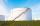The underground oil tank can be filled by two oil pipelines. The first is filled in 72 hours and the second in 48 hours. How many hours from the moment when first pipeline began to fill the oil is it necessary to start filling it with the second to fill i
• Water reservoir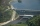One drain hole emptied the water from the tank for 20 hours, with the second drain for 30 hours. How long will the water drain from this tank if we open two drains simultaneously?